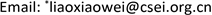Numerical Simulation Study of Incompletely Mixed Gas in Porous Media Combustion Flame Based on Venturi Mixture<sup> </sup>

Lin Zheng1, Xiaowei Liao2*, Hongtao Xu1, Feng Liu2

1School of Energy and Power Engineering, University of Shanghai for Science and Technology, Shanghai

2China Special Equipment Testing and Research Institute, BeijingReceived: Sep. 29th, 2020; accepted: Jan. 24th, 2021; published: Feb. 3rd, 2021ABSTRACT

The fuel/air Venturi mixer is widely used in the industrial premixed combustion. The uniformity of the mixed gas has a great influence on the combustion performance. In order to study the combustion characteristics of non-uniform mixed gas in porous media, this paper studied the methane/air non-uniform premixed gas after Venturi mixing and the Spatial Mixing Deficiency (SMD) is about 2%, when entering in the double-layer porous media burner. The research results show that: due to the influence of uneven mixing, non-premixed gas easily leads to local high temperature when ignited and burned in the porous medium and reduces the service life of the burner. When stable combustion is achieved, due to the good thermal conductivity of porous medium, the local high temperature can be flattened, but flame stability becomes worse.

Keywords:Incomplete Premixing, Porous Media, Combustion Temperature, Numerical Simulation1. 引言

2. 数值模拟2.1. 物理模型

Thermo-physical properties of Al2O3 and Si

2.2. 计算方法

1) 假设气体为不可压缩流体；

2) 多孔介质骨架为灰体且各向同性；

3) 多孔介质对燃烧反应没有催化作用并忽略由于多孔介质对气体的阻挡而产生的扰动对甲烷和空气产生的混和，既忽略弥散效应；

4) 忽略重力对气体的作用；

5) 参与燃烧反应的各组分以及燃烧产物为不可压缩理想气体。

6) 气体混合物在燃烧过程中各组分的化学性质(活化能Ea，指前因子等)不变。

1) 连续性方程

∂ ( ε ρ g ) ∂ t + ∇ ⋅ ( ε ρ g v ) = 0 (1)

2) 动量守恒方程

∂ ( ε ρ g v v ) ∂ t + ∇ ⋅ ( ε ρ g v v ) = − ∇ p + ∇ ⋅ ( μ ∇ v ) + f − R p (2)

R p = μ α v + C 2 ρ g 2 | v | v (3)

R p = A μ ( 1 − ε ) 2 D p 2 ε 3 v + 2 B ( 1 − ε ) D p ε 3 ρ g | v | v (4)

(1) 组分输运方程

∂ ( ε ρ g Y i ) ∂ t + ∇ ⋅ ( ε ρ g v Y i ) = ∇ ⋅ ( ε ρ g V i Y i ) + ε ω ˙ W i (5)

3) 能量方程

∂ ( ( ε ρ c p ) g + ( ( 1 − ε ) ρ c p ) s ) T ∂ t + ( ρ c p ) g ∇ ⋅ ( ε v T ) + ( ρ c p ) s ∇ ⋅ [ ( 1 − ε ) v T ] = ∇ ⋅ ( λ e f f ∇ T ) + ε ∑ i = 1 i ω ˙ i M i h i (6)

4) 理想气体状态方程

p V = n R T (7)

2.3. 数值方法

2.4. 参数计算

CH 4 + 2 L O 2 + 7.52 L N 2 = CO 2 + 2H 2 O + ( 2 L − 2 ) O 2 + 7.52 L N 2 (8)

η = 1 1 + 9.52 ∗ L (9)

v c h 4 = V i n A c h 4 = v A η A c h 4 (10)

v a i r = V i n A a i r = v A η A a i r (11)

SMD = ∬ | c − c ¯ | d A c ¯ A × 100 % (12)

2.5. 边界条件

λ e f f ∂ T s ∂ x = − ε σ ( T 4 − T 0 4 )

3. 计算结果及分析3.1. 文丘里混合性能分析

3.2. 非均匀预混多孔介质燃烧

4. 结论

1) 受到甲烷混合不均匀性的影响，非完全预混气体在多孔介质燃烧器中从点火开始到稳定燃烧的过程中容易产生局部高温而降低燃烧器的使用寿命。

2) 在达到稳定燃烧时，完全预混气体可以在多孔介质燃烧器内稳定燃烧，而预混不均匀使得非完全预混气体在多孔介质内的燃烧稳定性变差，其燃烧火焰发生了明显倾斜和弯曲。